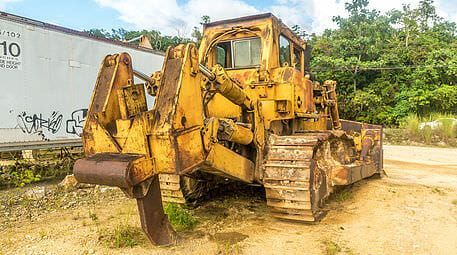# Depreciation Expense

Used to match expense of PP&E with its revenue generation

## What is Depreciation Expense?

When a long-term asset is purchased, it is often capitalized instead of being expensed in the given accounting period it is purchased in. This is because the asset will typically be economically useful and generate returns beyond that accounting period, so expensing it in that period would overstate the expense in that period and understate it in all future periods. To avoid this, depreciation is used to better match the expense of a long-term asset to the revenue it generates.

There are different methods used to calculate depreciation, and the type of depreciation accounting used is generally selected to match the nature of the equipment. For example, for vehicles that depreciate much faster in the first few years, an accelerated depreciation method is often chosen.

### Example of Depreciated Asset### What are the Depreciation Expense Methods?

There are three basic methods of calculating depreciation for a company:

1. Straight-line depreciation
2. Declining Balance (accelerated depreciation)
3. Units-of-production

#### #1 Straight-line depreciation

This is the most commonly used method of depreciation and is also the easiest to calculate. This method simply takes an equal depreciation expense each year, over the useful life of the asset.

Periodic Depreciation Expense = (Fair Value – Residual Value) / Useful life of Asset

For example, Company A purchases a building for \$50,000,000, to be used over 25 years, with no residual value. The annual depreciation expense is \$2,000,000, which is found by dividing \$50,000,000 by 25.

#### #2 Declining Balance

A declining balance depreciation is used when the asset depreciates faster in earlier years. As the name implies, the depreciation expense declines over time. To do this, the accountant picks a factor higher than one. In straight-line depreciation, the expense is found by multiplying the fair value with 1 / useful life. With the declining balance method, the factor can be 1.5, 2 or more. A 2x factor declining balance is known as a double declining balance depreciation schedule. As this is a popular option with accelerated depreciation schedules, it is often referred to as the “double declining balance” method.

Periodic Depreciation Expense = Beginning Value of Asset * Factor / Useful Life

The depreciation expense changes every year, because it is multiplied with the beginning value of the asset, which decreases over time due to accumulated depreciation.

For example, Company A has a vehicle worth \$100,000, with a useful life of 5 years. They want to depreciate with the double-declining balance. In the first year, the depreciated expense is \$40,000 (\$100,000 * 2 / 5). In the next year, the depreciation expense will be \$24,000 ( (\$100,000 – \$40,000) * 2 / 5).

See how the declining balance method is used in our financial modelling course.

#### #3 Units-of-Production

Under this method, the depreciation expense per unit produced is found by dividing the fair value, less residual value, of the asset by its useful life in terms of units, or the number of products, that it can be used to manufacture. This method sets a higher expense when production is higher, to match the higher usage of the equipment. It is, obviously, most useful for production machinery.

Unit Depreciation Expense = (Fair Value – Residual Value) / Useful Life in Units

Periodic Depreciation Expense = Unit Depreciation Expense * Units Produced

For example, Company A has a machine worth \$100,000, with a residual value of \$5,000. Production of units is 95,000. Thus, on a unit basis, the expense is (\$100,000 – \$5,000) / 95,000 = \$1. In one year, company A produces 10,000 units and, thus, records a depreciation expense of \$10,000

The units-of-production method is often used in mining operations. Check out our financial modeling course specialized for the mining industry.

Thank you for reading CFI’s explanation of how depreciation is calculated. To keep learning and developing your career, please check out these additional, relevant CFI resources:

• Debt Schedule
• Bonds Payables
• Accounts Payables
• Share Capital